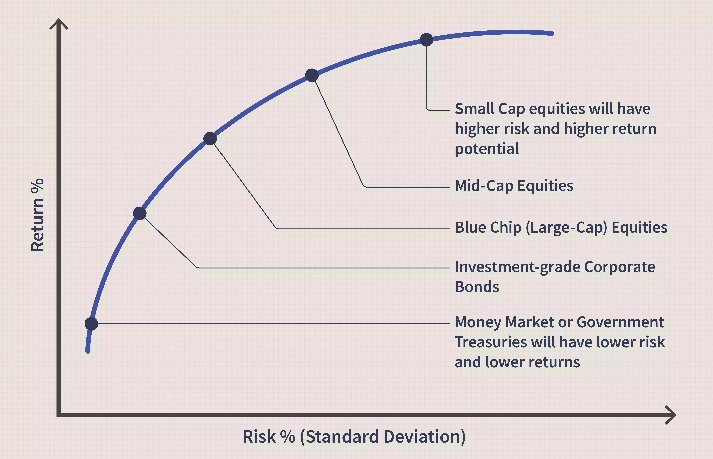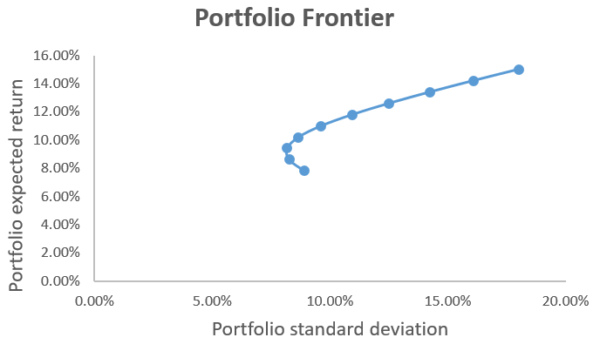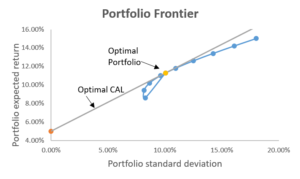Category: Investment Management

## What are the different strategies used to find out the optimum portfolio for an investor?

Stock investors constantly hear about the wisdom of diversification. This simply means don't put all your eggs in one basket. Those eggs are your money, and the baskets are the various asset classes you can choose to invest that money in. Deciding how many eggs or Rupees go into each basket is called asset allocation. This diversification helps reduce risk and generally leads to a better return on investment. The common wisdom in investing also tells us that we should set a target asset allocation in our portfolios and periodically rebalance to ensure our portfolio stays in line with our allocation goal. But does this make sense always? While the reasoning behind rebalancing is sound, it might lead to lower returns and higher commission costs. On the other side, what is the best portfolio balance which can optimize my return and risk, how can I find an optimal portfolio?

## Asset allocation

Asset allocation means spreading your investments across various asset classes. Broadly speaking, that means a mix of stocks, bonds, and cash or money market securities. This aims to control risk by diversifying an investment portfolio.

The goal of allocating your assets is to minimize risk while meeting the level of return you expect. To achieve that goal, you need to know the risk-return characteristics of the various asset classes. The figure below compares the risk and potential return of some of them:Source: Investopedia

Equities have the highest potential return but also the highest risk. Treasury bills have the lowest risk because they are backed by the central government, but they also provide the lowest return.

As each asset class has its level of return and risk, investors should consider their risk tolerance, investment objectives, time horizon, and available money to invest as the basis for their asset composition. All of this is important as investors look to create their optimal portfolio.

## Portfolio optimization

Portfolio optimization is the process of selecting the best portfolio (asset distribution), out of the set of all portfolios being considered, according to some objective. The objective typically maximizes factors such as expected return and minimizes costs like financial risk.

## What is an optimal portfolio?

An optimal portfolio is one that minimizes your risk for a given level of returns or maximizes your return for a given level of risk. What it means is that risk and returns cannot be seen in isolation. You need to take on a higher risk to earn higher returns. If you look at the graph above, there is a clear positive relationship between risk and return. The higher the risk taken, the higher is the return expectation and vice versa. When you are selecting a portfolio for yourself, you always try to ensure that your portfolio lies along the frontier. If your portfolio is below the curve, then it means you are not getting an adequate return for the risk taken. If it is above the curve, then it means you are getting more returns than justified by risk. This kind of situation is not sustainable, and you need to be wary of such portfolios.

## How to find an optimal portfolio?

It depends on what optimum means to you, risk and return are two aspects of investment, optimum means a balancing portfolio in terms of minimizing risk and maximizing return. There are different ways of finding an optimal portfolio. The commonly used and most preferred one is the Modern Portfolio Theory (MPT) put forward by Harry Markowitz. The theory says that there is no such thing as the perfect investment but crafting a strategy that offers high returns and relatively low risk is the priority for modern investors.

Instead of focusing on the risk of each asset, Markowitz demonstrated that a diversified portfolio is less volatile than the total sum of individual assets. While each asset itself might be quite volatile, the volatility of the entire portfolio can be quite low.

This theory was based on two main concepts:

1. Every investor’s goal is to maximize return for any level of risk

2. Risk can be reduced by diversifying a portfolio through individual, unrelated securities

MPT works under the assumption that investors are risk-averse, preferring a portfolio with less risk for a given level of return. Under this assumption, investors will only take on high-risk investments if they can expect a larger reward.

## How does this theory work?

For the sake of simplicity, we will construct a portfolio with only two risky assets.

The portfolio’s expected return is a weighted average of its individual assets’ expected returns, and is calculated as:

E(Rp) = w1E(R1) + w2E(R2)

Where w1, w2 are the respective weights for the two assets, and E(R1), E(R2) are the respective expected returns.

Levels of variance translate directly with levels of risk, higher variance means higher levels of risk, and vice versa. The variance of a portfolio is not just the weighted average of the variance of individual assets but also depends on the covariance and correlation of the two assets. The formula for portfolio variance is given as:

Var(Rp) = w21Var(R1) + w22Var(R2) + 2w1w2Cov(R1, R2)

Where Cov(R1, R2) represents the covariance of the two asset returns.

The variance of portfolio return is greater when the covariance of the two assets is positive, and less when negative. Since variance represents a risk, the portfolio risk is lower when its asset components possess negative covariance. Diversification is a technique that minimizes portfolio risk by investing in assets with negative covariance.

To construct a portfolio frontier, we first assign values for E(R1), E(R2), stdev(R1), stdev(R2), and ρ(R1, R2). Using the above formulas, we then calculate the portfolio expected return and variance for each possible asset weight combinations (w2=1-w1). This will be plot into a scatter chart with smooth lines. The graph will look like below.According to the mean-variance criterion, any investor would optimally select a portfolio on the upward-sloping portion of the portfolio frontier, which is called the efficient frontier, or minimum variance frontier. The choice of any portfolio on the efficient frontier depends on the investor’s risk preferences.

## Capital Allocation Line (CAL)

The capital allocation line (CAL), also known as the capital market link (CML), is a line created on a graph of all possible combinations of risk-free and risky assets. The graph displays the return investors might earn by assuming a certain level of risk with their investment. The slope of the CAL is known as the reward-to-variability ratio.

## Optimal Portfolio

The optimal portfolio consists of a risk-free asset and an optimal risky asset portfolio. The optimal risky asset portfolio is at the point where the CAL is tangent to the efficient frontier. This portfolio is optimal because the slope of CAL is the highest, which means we achieve the highest returns per additional unit of risk.Source: CFI

## Conclusion

Asset allocation is a fundamental investing principle that helps investors maximize profits while minimizing risk. Once you have chosen an asset allocation strategy that's right for you, remember to review your portfolio periodically to ensure that you're maintaining your intended allocation and are still on track to your long-term investment goals.

Ranjith

Dilzer Consultants Pvt Ltd

Reference:

https://www.investopedia.com/articles/basics/11/5-popular-portfolio-types.asp

https://investresolve.com/blog/portfolio-optimization-simple-optimal-methods/

https://corporatefinanceinstitute.com/resources/knowledge/finance/capital-allocation-line-cal-and-optimal-portfolio/

https://www.researchgate.net/publication/308617229_Improving_the_Naive_Portfolio_Strategy

https://www.bis.org/publ/bppdf/bispap58i.pdf

http://people.brandeis.edu/~danielak/Out%20of%20sample%20performance%20of%20asset%20allocation%20strategies.pdf

abcd